1620177818

# Django admin full Customization step by step

Welcome to my blog , hey everyone in this article you learn how to customize the Django app and view in the article you will know how to register  and unregister  models from the admin view how to add filtering how to add a custom input field, and a button that triggers an action on all objects and even how to change the look of your app and page using the Django suit package let’s get started.

## Buddha Community1620177818

## Django admin full Customization step by step

Welcome to my blog , hey everyone in this article you learn how to customize the Django app and view in the article you will know how to register  and unregister  models from the admin view how to add filtering how to add a custom input field, and a button that triggers an action on all objects and even how to change the look of your app and page using the Django suit package let’s get started.

### Custom Titles of Django Admin1561523460

## Matplotlib Cheat Sheet: Plotting in Python

This Matplotlib cheat sheet introduces you to the basics that you need to plot your data with Python and includes code samples.

Data visualization and storytelling with your data are essential skills that every data scientist needs to communicate insights gained from analyses effectively to any audience out there.

For most beginners, the first package that they use to get in touch with data visualization and storytelling is, naturally, Matplotlib: it is a Python 2D plotting library that enables users to make publication-quality figures. But, what might be even more convincing is the fact that other packages, such as Pandas, intend to build more plotting integration with Matplotlib as time goes on.

However, what might slow down beginners is the fact that this package is pretty extensive. There is so much that you can do with it and it might be hard to still keep a structure when you're learning how to work with Matplotlib.

DataCamp has created a Matplotlib cheat sheet for those who might already know how to use the package to their advantage to make beautiful plots in Python, but that still want to keep a one-page reference handy. Of course, for those who don't know how to work with Matplotlib, this might be the extra push be convinced and to finally get started with data visualization in Python.

You'll see that this cheat sheet presents you with the six basic steps that you can go through to make beautiful plots.

Check out the infographic by clicking on the button below: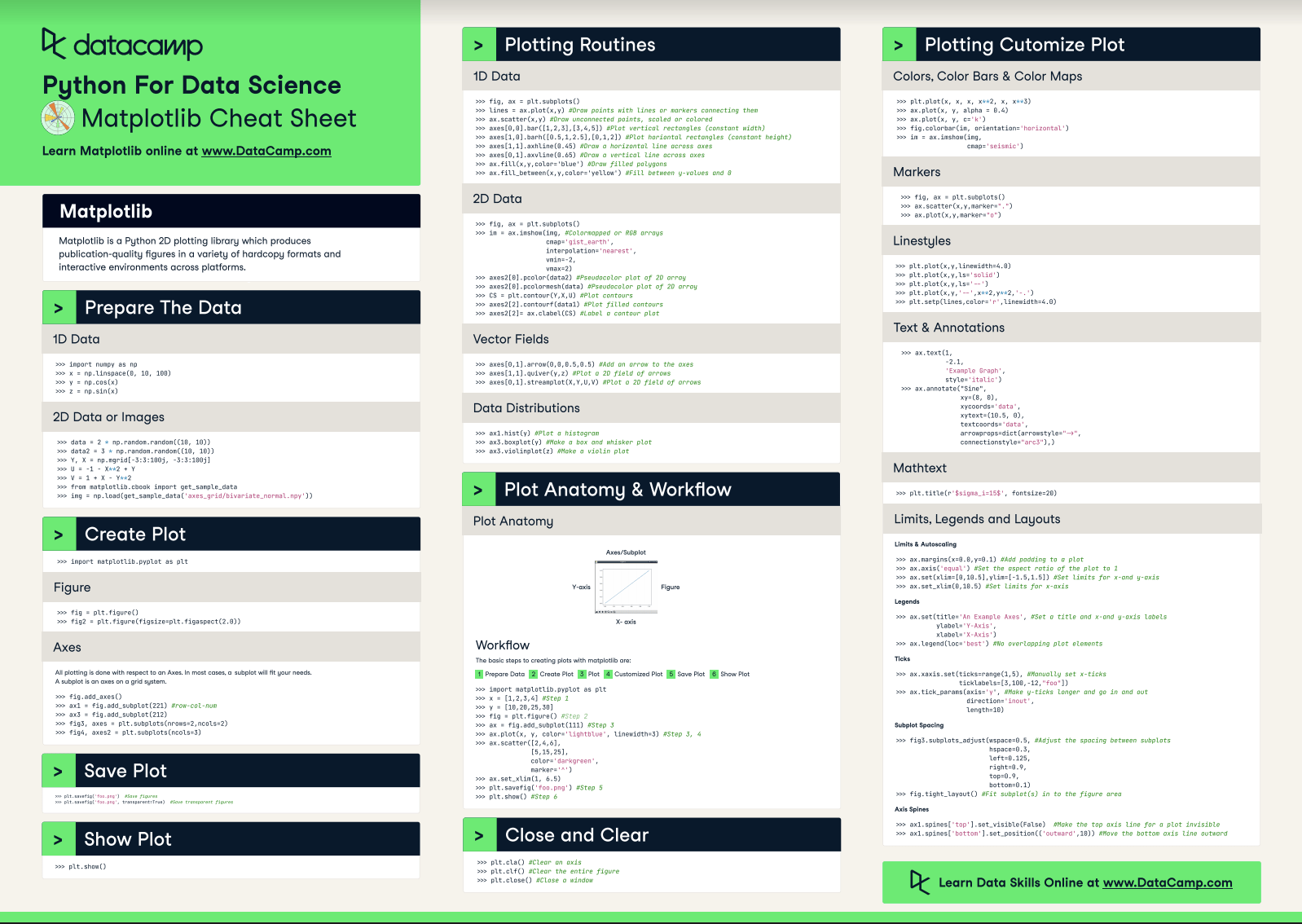With this handy reference, you'll familiarize yourself in no time with the basics of Matplotlib: you'll learn how you can prepare your data, create a new plot, use some basic plotting routines to your advantage, add customizations to your plots, and save, show and close the plots that you make.

What might have looked difficult before will definitely be more clear once you start using this cheat sheet! Use it in combination with the Matplotlib Gallery, the documentation.

Matplotlib

Matplotlib is a Python 2D plotting library which produces publication-quality figures in a variety of hardcopy formats and interactive environments across platforms.

## Prepare the Data

### 1D Data

``````>>> import numpy as np
>>> x = np.linspace(0, 10, 100)
>>> y = np.cos(x)
>>> z = np.sin(x)``````

### 2D Data or Images

``````>>> data = 2 * np.random.random((10, 10))
>>> data2 = 3 * np.random.random((10, 10))
>>> Y, X = np.mgrid[-3:3:100j, -3:3:100j]
>>> U = 1 X** 2 + Y
>>> V = 1 + X Y**2
>>> from matplotlib.cbook import get_sample_data

## Create Plot

``>>> import matplotlib.pyplot as plt``

### Figure

``````>>> fig = plt.figure()
>>> fig2 = plt.figure(figsize=plt.figaspect(2.0))``````

### Axes

``````>>> fig.add_axes()
>>> fig3, axes = plt.subplots(nrows=2,ncols=2)
>>> fig4, axes2 = plt.subplots(ncols=3)``````

## Save Plot

``````>>> plt.savefig('foo.png') #Save figures
>>> plt.savefig('foo.png',  transparent=True) #Save transparent figures``````

## Show Plot

``>>> plt.show()``

## 1D Data

``````>>> fig, ax = plt.subplots()
>>> lines = ax.plot(x,y) #Draw points with lines or markers connecting them
>>> ax.scatter(x,y) #Draw unconnected points, scaled or colored
>>> axes[0,0].bar([1,2,3],[3,4,5]) #Plot vertical rectangles (constant width)
>>> axes[1,0].barh([0.5,1,2.5],[0,1,2]) #Plot horiontal rectangles (constant height)
>>> axes[1,1].axhline(0.45) #Draw a horizontal line across axes
>>> axes[0,1].axvline(0.65) #Draw a vertical line across axes
>>> ax.fill(x,y,color='blue') #Draw filled polygons
>>> ax.fill_between(x,y,color='yellow') #Fill between y values and 0``````

### 2D Data

``````>>> fig, ax = plt.subplots()
>>> im = ax.imshow(img, #Colormapped or RGB arrays
cmap= 'gist_earth',
interpolation= 'nearest',
vmin=-2,
vmax=2)
>>> axes2.pcolor(data2) #Pseudocolor plot of 2D array
>>> axes2.pcolormesh(data) #Pseudocolor plot of 2D array
>>> CS = plt.contour(Y,X,U) #Plot contours
>>> axes2.contourf(data1) #Plot filled contours
>>> axes2= ax.clabel(CS) #Label a contour plot``````

### Vector Fields

``````>>> axes[0,1].arrow(0,0,0.5,0.5) #Add an arrow to the axes
>>> axes[1,1].quiver(y,z) #Plot a 2D field of arrows
>>> axes[0,1].streamplot(X,Y,U,V) #Plot a 2D field of arrows``````

### Data Distributions

``````>>> ax1.hist(y) #Plot a histogram
>>> ax3.boxplot(y) #Make a box and whisker plot
>>> ax3.violinplot(z)  #Make a violin plot``````

## Plot Anatomy & Workflow

### Plot Anatomy

y-axis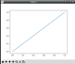x-axis

### Workflow

The basic steps to creating plots with matplotlib are:

1 Prepare Data
2 Create Plot
3 Plot
4 Customized Plot
5 Save Plot
6 Show Plot

``````>>> import matplotlib.pyplot as plt
>>> x = [1,2,3,4]  #Step 1
>>> y = [10,20,25,30]
>>> fig = plt.figure() #Step 2
>>> ax = fig.add_subplot(111) #Step 3
>>> ax.plot(x, y, color= 'lightblue', linewidth=3)  #Step 3, 4
>>> ax.scatter([2,4,6],
[5,15,25],
color= 'darkgreen',
marker= '^' )
>>> ax.set_xlim(1, 6.5)
>>> plt.savefig('foo.png' ) #Step 5
>>> plt.show() #Step 6``````

## Close and Clear

``````>>> plt.cla()  #Clear an axis
>>> plt.clf(). #Clear the entire figure
>>> plt.close(). #Close a window``````

## Plotting Customize Plot

### Colors, Color Bars & Color Maps

``````>>> plt.plot(x, x, x, x**2, x, x** 3)
>>> ax.plot(x, y, alpha = 0.4)
>>> ax.plot(x, y, c= 'k')
>>> fig.colorbar(im, orientation= 'horizontal')
>>> im = ax.imshow(img,
cmap= 'seismic' )``````

### Markers

``````>>> fig, ax = plt.subplots()
>>> ax.scatter(x,y,marker= ".")
>>> ax.plot(x,y,marker= "o")``````

### Linestyles

``````>>> plt.plot(x,y,linewidth=4.0)
>>> plt.plot(x,y,ls= 'solid')
>>> plt.plot(x,y,ls= '--')
>>> plt.plot(x,y,'--' ,x**2,y**2,'-.' )
>>> plt.setp(lines,color= 'r',linewidth=4.0)``````

### Text & Annotations

``````>>> ax.text(1,
-2.1,
'Example Graph',
style= 'italic' )
>>> ax.annotate("Sine",
xy=(8, 0),
xycoords= 'data',
xytext=(10.5, 0),
textcoords= 'data',
arrowprops=dict(arrowstyle= "->",
connectionstyle="arc3"),)``````

### Mathtext

``>>> plt.title(r '\$sigma_i=15\$', fontsize=20)``

### Limits, Legends and Layouts

Limits & Autoscaling

``````>>> ax.margins(x=0.0,y=0.1) #Add padding to a plot
>>> ax.axis('equal')  #Set the aspect ratio of the plot to 1
>>> ax.set(xlim=[0,10.5],ylim=[-1.5,1.5])  #Set limits for x-and y-axis
>>> ax.set_xlim(0,10.5) #Set limits for x-axis``````

Legends

``````>>> ax.set(title= 'An Example Axes',  #Set a title and x-and y-axis labels
ylabel= 'Y-Axis',
xlabel= 'X-Axis')
>>> ax.legend(loc= 'best')  #No overlapping plot elements``````

Ticks

``````>>> ax.xaxis.set(ticks=range(1,5),  #Manually set x-ticks
ticklabels=[3,100, 12,"foo" ])
>>> ax.tick_params(axis= 'y', #Make y-ticks longer and go in and out
direction= 'inout',
length=10)``````

Subplot Spacing

``````>>> fig3.subplots_adjust(wspace=0.5,   #Adjust the spacing between subplots
hspace=0.3,
left=0.125,
right=0.9,
top=0.9,
bottom=0.1)
>>> fig.tight_layout() #Fit subplot(s) in to the figure area``````

Axis Spines

``````>>> ax1.spines[ 'top'].set_visible(False) #Make the top axis line for a plot invisible
>>> ax1.spines['bottom' ].set_position(( 'outward',10))  #Move the bottom axis line outward``````

Have this Cheat Sheet at your fingertips

Original article source at https://www.datacamp.com

#matplotlib #cheatsheet #python1620185280

## How model queries work in Django

**Read More : **How to make Chatbot in Python.

let’s just get into this diagram that I made so in here:Describe each parameter in Django querset

we’re making a simple query for the myModel table so we want to pull out all the information in the database so we have this variable which is gonna hold a return value and we have our myModel models so this is simply the myModel model name so whatever you named your model just make sure you specify that and we’re gonna access the objects attribute once we get that object’s attribute we can simply use the all method and this will return all the information in the database so we’re gonna start with all and then we will go into getting single items filtering that data and go to our command prompt.

Here and we’ll actually start making our queries from here to do this let’s just go ahead and run** Python manage.py shell** and I am in my project file so make sure you’re in there when you start and what this does is it gives us an interactive shell to actually start working with our data so this is a lot like the Python shell but because we did manage.py it allows us to do things a Django way and actually query our database now open up the command prompt and let’s go ahead and start making our first queries.

#django #django model queries #django orm #django queries #django query #model django query #model query #query with django1653464648

## Python Data Visualization: Bokeh Cheat Sheet

A handy cheat sheet for interactive plotting and statistical charts with Bokeh.

Bokeh distinguishes itself from other Python visualization libraries such as Matplotlib or Seaborn in the fact that it is an interactive visualization library that is ideal for anyone who would like to quickly and easily create interactive plots, dashboards, and data applications.

Bokeh is also known for enabling high-performance visual presentation of large data sets in modern web browsers.

For data scientists, Bokeh is the ideal tool to build statistical charts quickly and easily; But there are also other advantages, such as the various output options and the fact that you can embed your visualizations in applications. And let's not forget that the wide variety of visualization customization options makes this Python library an indispensable tool for your data science toolbox.

Now, DataCamp has created a Bokeh cheat sheet for those who have already taken the course and that still want a handy one-page reference or for those who need an extra push to get started.

In short, you'll see that this cheat sheet not only presents you with the five steps that you can go through to make beautiful plots but will also introduce you to the basics of statistical charts.

In no time, this Bokeh cheat sheet will make you familiar with how you can prepare your data, create a new plot, add renderers for your data with custom visualizations, output your plot and save or show it. And the creation of basic statistical charts will hold no secrets for you any longer.

Boost your Python data visualizations now with the help of Bokeh! :)

## Plotting With Bokeh

The Python interactive visualization library Bokeh enables high-performance visual presentation of large datasets in modern web browsers.

Bokeh's mid-level general-purpose bokeh. plotting interface is centered around two main components: data and glyphs.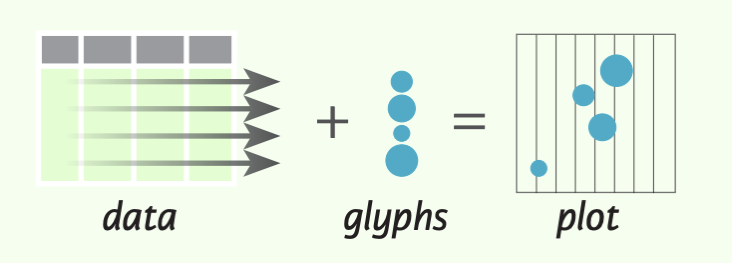The basic steps to creating plots with the bokeh. plotting interface are:

1. Prepare some data (Python lists, NumPy arrays, Pandas DataFrames and other sequences of values)
2. Create a new plot
4. Specify where to generate the output
5. Show or save the results
``````>>> from bokeh.plotting import figure
>>> from bokeh.io import output_file, show
>>> x = [1, 2, 3, 4, 5] #Step 1
>>> y = [6, 7, 2, 4, 5]
>>> p = figure(title="simple line example", #Step 2
x_axis_label='x',
y_axis_label='y')
>>> p.line(x, y, legend="Temp.", line_width=2) #Step 3
>>> output_file("lines.html") #Step 4
>>> show(p) #Step 5``````

## 1. Data

Under the hood, your data is converted to Column Data Sources. You can also do this manually:

``````>>> import numpy as np
>>> import pandas as pd
>>> df = pd.OataFrame(np.array([[33.9,4,65, 'US'], [32.4, 4, 66, 'Asia'], [21.4, 4, 109, 'Europe']]),
columns= ['mpg', 'cyl',   'hp',   'origin'],
index=['Toyota', 'Fiat', 'Volvo'])

>>> from bokeh.models import ColumnOataSource
>>> cds_df = ColumnOataSource(df)``````

## 2. Plotting

``````>>> from bokeh.plotting import figure
>>>p1= figure(plot_width=300, tools='pan,box_zoom')
>>> p2 = figure(plot_width=300, plot_height=300,
x_range=(0, 8), y_range=(0, 8))
>>> p3 = figure()``````

## 3. Renderers & Visual Customizations

### Glyphs

Scatter Markers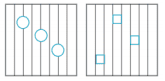``````>>> p1.circle(np.array([1,2,3]), np.array([3,2,1]), fill_color='white')
>>> p2.square(np.array([1.5,3.5,5.5]), [1,4,3],
color='blue', size=1)
``````

Line Glyphs``````>>> pl.line([1,2,3,4], [3,4,5,6], line_width=2)
>>> p2.multi_line(pd.DataFrame([[1,2,3],[5,6,7]]),
pd.DataFrame([[3,4,5],[3,2,1]]),
color="blue")
``````

### Customized Glyphs

Selection and Non-Selection Glyphs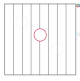``````>>> p = figure(tools='box_select')
>>> p. circle ('mpg', 'cyl', source=cds_df,
selection_color='red',
nonselection_alpha=0.1)
``````

Hover Glyphs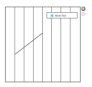``````>>> from bokeh.models import HoverTool
>>>hover= HoverTool(tooltips=None, mode='vline')
``````

Color Mapping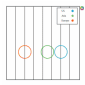``````>>> from bokeh.models import CategoricalColorMapper
>>> color_mapper = CategoricalColorMapper(
factors= ['US', 'Asia', 'Europe'],
palette= ['blue', 'red', 'green'])
>>>  p3. circle ('mpg', 'cyl', source=cds_df,
color=dict(field='origin',
transform=color_mapper), legend='Origin')
``````

## 4. Output & Export

### Notebook

``````>>> from bokeh.io import output_notebook, show
>>> output_notebook()
``````

### HTML

Standalone HTML

``````>>> from bokeh.embed import file_html
>>> from bokeh.resources import CON
>>> html = file_html(p, CON, "my_plot")

>>> from  bokeh.io  import  output_file,  show
>>> output_file('my_bar_chart.html',  mode='cdn')``````

Components

``````>>> from bokeh.embed import components
>>> script, div= components(p)``````

### PNG

``````>>> from bokeh.io import export_png
>>> export_png(p, filename="plot.png")``````

### SVG

``````>>> from bokeh.io import export_svgs
>>> p. output_backend = "svg"
>>> export_svgs(p,filename="plot.svg")``````

### Legend Location

Inside Plot Area

``````>>> p.legend.location = 'bottom left'
``````

Outside Plot Area

``````>>> from bokeh.models import Legend
>>> r1 = p2.asterisk(np.array([1,2,3]), np.array([3,2,1])
>>> r2 = p2.line([1,2,3,4], [3,4,5,6])
>>> legend = Legend(items=[("One" ,[p1, r1]),("Two",[r2])], location=(0, -30))

### Legend Background & Border

``````>>> p.legend. border_line_color = "navy"
>>> p.legend.background_fill_color = "white"``````

### Legend Orientation

``````>>> p.legend.orientation = "horizontal"
>>> p.legend.orientation = "vertical"``````

### Rows & Columns Layout

Rows

``````>>> from bokeh.layouts import row
>>>layout= row(p1,p2,p3)
``````

Columns

``````>>> from bokeh.layouts import columns
>>>layout= column(p1,p2,p3)``````

Nesting Rows & Columns

``````>>>layout= row(column(p1,p2), p3)
``````

### Grid Layout

``````>>> from bokeh.layouts import gridplot
>>> rowl = [p1,p2]
>>> row2 = [p3]
>>> layout = gridplot([[p1, p2],[p3]])``````

### Tabbed Layout

``````>>> from bokeh.models.widgets import Panel, Tabs
>>> tab1 = Panel(child=p1, title="tab1")
>>> tab2 = Panel(child=p2, title="tab2")
>>> layout = Tabs(tabs=[tab1, tab2])``````

``````Linked Axes
>>> p2.x_range = p1.x_range
>>> p2.y_range = p1.y_range``````

``````>>> p4 = figure(plot_width = 100, tools='box_select,lasso_select')
>>> p4.circle('mpg', 'cyl' , source=cds_df)
>>> p5 = figure(plot_width = 200, tools='box_select,lasso_select')
>>> p5.circle('mpg', 'hp', source=cds df)
>>>layout= row(p4,p5)``````

## 5. Show or Save Your Plots

``````>>> show(p1)
>>> show(layout)
>>> save(p1)``````

Have this Cheat Sheet at your fingertips

Original article source at https://www.datacamp.com

#python #datavisualization #bokeh #cheatsheet1645619585

## Django Chartit: A Django App to Plot Charts and Pivot Charts Directly

Django Chartit is a Django app that can be used to easily create charts from the data in your database. The charts are rendered using `Highcharts` and `jQuery` JavaScript libraries. Data in your database can be plotted as simple line charts, column charts, area charts, scatter plots, and many more chart types. Data can also be plotted as Pivot Charts where the data is grouped and/or pivoted by specific column(s).

## Features

• Plot charts from models.
• Plot data from multiple models on the same axis on a chart.
• Plot pivot charts from models. Data can be pivoted by across multiple columns.
• Legend pivot charts by multiple columns.
• Combine data from multiple models to plot on same pivot charts.
• Plot a pareto chart, paretoed by a specific column.
• Plot only a top few items per category in a pivot chart.
• Python 3 compatibility
• Django 1.8 and 1.9 compatibility
• Automated testing via Travis CI
• Test coverage tracking via Coveralls

## Installation

You can install Django-Chartit from PyPI. Just do

``````\$ pip install django_chartit
``````

Then, add chartit to INSTALLED_APPS in "settings.py".

You also need supporting JavaScript libraries. See the Required JavaScript Libraries section for more details.

## How to Use

Plotting a chart or pivot chart on a webpage involves the following steps.

1. Create a `DataPool` or `PivotDataPool` object that specifies what data you need to retrieve and from where.
2. Create a `Chart` or `PivotChart` object to plot the data in the `DataPool` or `PivotDataPool` respectively.
3. Return the `Chart`/`PivotChart` object from a django `view` function to the django template.
4. Use the `load_charts` template tag to load the charts to HTML tags with specific ids.

It is easier to explain the steps above with examples. So read on.

## How to Create Charts

Here is a short example of how to create a line chart. Let's say we have a simple model with 3 fields - one for month and two for temperatures of Boston and Houston.

``````class MonthlyWeatherByCity(models.Model):
month = models.IntegerField()
boston_temp = models.DecimalField(max_digits=5, decimal_places=1)
houston_temp = models.DecimalField(max_digits=5, decimal_places=1)
``````

And let's say we want to create a simple line chart of month on the x-axis and the temperatures of the two cities on the y-axis.

``````from chartit import DataPool, Chart

def weather_chart_view(request):
#Step 1: Create a DataPool with the data we want to retrieve.
weatherdata = \
DataPool(
series=
[{'options': {
'source': MonthlyWeatherByCity.objects.all()},
'terms': [
'month',
'houston_temp',
'boston_temp']}
])

#Step 2: Create the Chart object
cht = Chart(
datasource = weatherdata,
series_options =
[{'options':{
'type': 'line',
'stacking': False},
'terms':{
'month': [
'boston_temp',
'houston_temp']
}}],
chart_options =
{'title': {
'text': 'Weather Data of Boston and Houston'},
'xAxis': {
'title': {
'text': 'Month number'}}})

#Step 3: Send the chart object to the template.
return render_to_response({'weatherchart': cht})
``````

And you can use the `load_charts` filter in the django template to render the chart.

``````<head>
<!-- code to include the highcharts and jQuery libraries goes here -->
<!-- load_charts filter takes a comma-separated list of id's where -->
<!-- the charts need to be rendered to                             -->
<body>
<div id='container'> Chart will be rendered here </div>
</body>
``````

## How to Create Pivot Charts

Here is an example of how to create a pivot chart. Let's say we have the following model.

``````class DailyWeather(models.Model):
month = models.IntegerField()
day = models.IntegerField()
temperature = models.DecimalField(max_digits=5, decimal_places=1)
rainfall = models.DecimalField(max_digits=5, decimal_places=1)
city = models.CharField(max_length=50)
state = models.CharField(max_length=2)
``````

We want to plot a pivot chart of month (along the x-axis) versus the average rainfall (along the y-axis) of the top 3 cities with highest average rainfall in each month.

``````from django.db.models import Avg
from chartit import PivotDataPool, PivotChart

def rainfall_pivot_chart_view(request):
# Step 1: Create a PivotDataPool with the data we want to retrieve.
rainpivotdata = PivotDataPool(
series=[{
'options': {
'source': DailyWeather.objects.all(),
'categories': ['month'],
'legend_by': 'city',
'top_n_per_cat': 3,
},
'terms': {
'avg_rain': Avg('rainfall'),
}
}]
)

# Step 2: Create the PivotChart object
rainpivcht = PivotChart(
datasource=rainpivotdata,
series_options=[{
'options': {
'type': 'column',
'stacking': True
},
'terms': ['avg_rain']
}],
chart_options={
'title': {
'text': 'Rain by Month in top 3 cities'
},
'xAxis': {
'title': {
'text': 'Month'
}
}
}
)

# Step 3: Send the PivotChart object to the template.
return render_to_response({'rainpivchart': rainpivcht})
``````

And you can use the `load_charts` filter in the django template to render the chart.

``````<head>
<!-- code to include the highcharts and jQuery libraries goes here -->
<!-- load_charts filter takes a comma-separated list of id's where -->
<!-- the charts need to be rendered to                             -->
<body>
<div id='container'> Chart will be rendered here </div>
</body>
``````

## Rendering multiple charts

It is possible to render multiple charts in the same template. The first argument to `load_charts` is the Chart object or a list of Chart objects, and the second is a comma separated list of HTML IDs where the charts will be rendered.

When calling Django's `render` you have to pass all you charts as a list:

``````return render(request, 'index.html',
{
'chart_list' : [chart_1, chart_2],
}
)
``````

Then in your template you have to use the proper syntax:

``````<head>
<body>
<div id="chart_1">First chart will be rendered here</div>
<div id="chart_2">Second chart will be rendered here</div>
</body>
``````

## Demo

The above examples are just a brief taste of what you can do with Django-Chartit. For more examples and to look at the charts in actions, check out the `demoproject/` directory. To execute the demo run the commands

``````cd demoproject/
PYTHONPATH=../ python ./manage.py migrate
PYTHONPATH=../ python ./manage.py runserver
``````

## Documentation

Full documentation is available here .

## Required JavaScript Libraries

The following JavaScript Libraries are required for using Django-Chartit.

• jQuery - versions 1.6.4 and 1.7 are known to work well with django-chartit.
• Highcharts - versions 2.1.7 and 2.2.0 are known to work well with django-chartit.

Note

While `Django-Chartit` itself is licensed under the BSD license, `Highcharts` is licensed under the Highcharts license and `jQuery` is licensed under both MIT License and GNU General Public License (GPL) Version 2. It is your own responsibility to abide by respective licenses when downloading and using the supporting JavaScript libraries.

0.1 (November 5, 2011)

• Initial release of django-chartit

0.2.0 as django-chartit2 (January 20, 2016):

• Fixed issue that could prevent installation via PyPI

0.2.2 as django-chartit2 (January 28, 2016)

• Fixed another issue that prevented installation via PyPI

0.2.3 (July 30, 2016)

• New to_json() method for charts. Useful for creating Highcharts in AJAX
• Merged with django-chartit2 fork by Grant McConnaughey which adds Python 3 and latest Django 1.8.x and 1.9.x support
• Allow dictionary fields in conjunction with lambda fields. Closes #26
• Documentation improvements
• Lots of code cleanups and style improvements

0.2.4 (August 2, 2016)

• Fix for `get_all_field_names()` and `get_field_by_name()` removal in Django 1.10. Fixes #39
• Updated for django.db.sql.query.Query.aggregates removal

0.2.5 (August 3, 2016)

• Workaround Python 3 vs. Python 2 list sort issue which breaks charts with multiple data sources displayed on the same axis!
• Make demoproject/ compatible with Django 1.10

0.2.6 (August 16, 2016)

• Merge `chartit_tests/` with `demoproject/`
• Load test DB with real data to use during testing
• Update the path to demoproject.settings when building docs. Fixes a problem which caused some API docs to be empty
• Fix ValueError: not enough values to unpack (expected 2, got 0) with PivotChart when the QuerySet returns empty data
• Dropped requirement on `simplejson`
• Properly handle unicode data in Pivot charts. Fixes #5
• Demo project updated with Chart and PivotChart examples of rendering DateField values on the X axis
• Allow charting of `extra()` or `annotate()` fields. Fixes #8 and #12
• Refactor `RecursiveDefaultDict` to allow chart objects to be serialized to/from cache. Fixes #10

0.2.7 (September 14, 2016)

• Don't use `super(self.__class__)` b/c that breaks chart class inheritance. Fixes #41

0.2.8 (December 4, 2016)

• `PivotChart` and `PivotDataPool` will be deprecated soon. Both are marked with deprecation warnings. There is a lot of duplication and special handling between those classes and the `Chart` and `DataPool` classes which make it harder to expand the feature set for django-chartit. The next release will focus on consolidating all the functionality into `Chart` and `DataPool` so that users will still be able to draw pivot charts. You will have to construct your pivot charts manually though!
• `DataPool` terms now supports model properties. Fixes #35. Model properties are not supported for `PivotDataPool`! WARNING: when using model properties chartit can't make use of ``QuerySet.values()`` internally. This means results will not be groupped by the values of the fields you supplied. This may lead to unexpected query results/charts!
• `DataPool` now supports `RawQuerySet` as data source. Fixes #44. `RawQuerySet` is not supported for `PivotDataPool`! WARNING: when using ``RawQuerySet`` don't use double underscores in field names because these are interpreted internally by chartit and will cause exceptions. For example don't do this ``SELECT AVG(rating) as rating__avg`` instead write it as ``SELECT AVG(rating) as rating_avg``!
• README now tells how to execute `demoproject/`

0.2.9 (January 17, 2017)

• Enable pylint during testing but don't block Travis-CI on failures. Closes #42.
• Handle unicode data in pie and scatter plot charts under Python 2.7. PR#47.

master

• Update demo with an example of how to pass `legendIndex` as an option to a data serie. Closes #48.
• Update demo with an example of how to change the label of any term instead of using the default one. Closes #46.